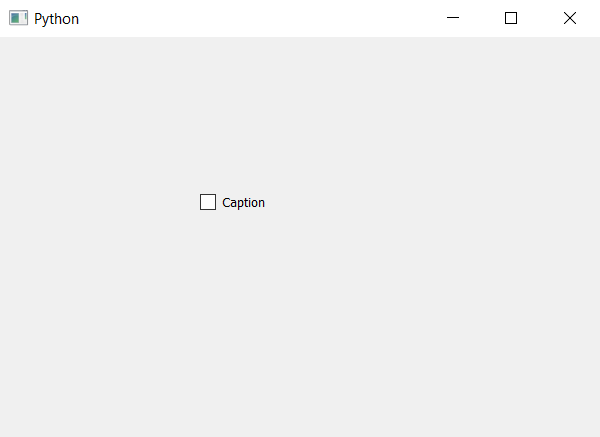# PyQt5 – Adding/Changing caption of Check Box

In this article we will see how to add or change the caption associated with the check box. In check box there are basically two parts one it the check part which consist of the checked symbol and other is the text part which is similar to the label it contain text.

In order to add or change the text of check box we will use `setText` method.

Syntax : checkbox.setText(caption)

Argument : It takes string as argument.

Action performed : It will replace the existing caption with new one

Below is the implementation.

 `# importing libraries ` `from` `PyQt5.QtWidgets ``import` `*`  `from` `PyQt5 ``import` `QtCore, QtGui ` `from` `PyQt5.QtGui ``import` `*`  `from` `PyQt5.QtCore ``import` `*`  `import` `sys ` ` `  ` `  `class` `Window(QMainWindow): ` ` `  `    ``def` `__init__(``self``): ` `        ``super``().__init__() ` ` `  `        ``# setting title ` `        ``self``.setWindowTitle(``"Python "``) ` ` `  `        ``# setting geometry ` `        ``self``.setGeometry(``100``, ``100``, ``600``, ``400``) ` ` `  `        ``# calling method ` `        ``self``.UiComponents() ` ` `  `        ``# showing all the widgets ` `        ``self``.show() ` ` `  `    ``# method for widgets ` `    ``def` `UiComponents(``self``): ` ` `  `        ``# creating the check-box ` `        ``checkbox ``=` `QCheckBox(``'Check Box'``, ``self``) ` ` `  `        ``# setting geometry of check box ` `        ``checkbox.setGeometry(``200``, ``150``, ``100``, ``30``) ` ` `  `        ``# setting check box state to checked ` `        ``checkbox.setText(``"Caption"``) ` ` `  ` `  `# create pyqt5 app ` `App ``=` `QApplication(sys.argv) ` ` `  `# create the instance of our Window ` `window ``=` `Window() ` ` `  `# start the app ` `sys.exit(App.``exec``()) `

Output :